×

Search anything:

# Worst Case of Merge Sort

#### Time Complexity Sorting Algorithms AlgorithmsGet this book -> Problems on Array: For Interviews and Competitive Programming

In this article, we have covered the scenario when Merge Sort performs worst, how to identify such worst case inputs and Time Complexity analysis of Worst Case of Merge Sort.

#### Content:

• Overview
• How it works
• When does Worst Case occus
• Worst Case Time complexity analysis of Merge Sort
• Implementation of Merge Sort
• Conclusion

In short, the worst case of Merge Sort is when the left and right array has alternating elements. This results in maximum number of comparison and the Time Complexity stays at O(N logN).

## Overview:

Merge Sort is one of the most efficient sorting algorithm which is based on divide and conquer approach. Basically in Merge Sort, we divide the imput array into 2 parts and then call itself to sort both parts via recursion and finally merge the both sorted parts. In Merge Sort after sorting two halves via recusrion we call merge function which merge the two sorted sub-arrays into one.

## How it works:

Lets discuss the working of Merge Sort in following steps:
Consider an array = {8,3,4,12,5,6}

1. First, we will divide the array into two halves by fining the middle index.
midIndex = (startIndex + endIndex)/2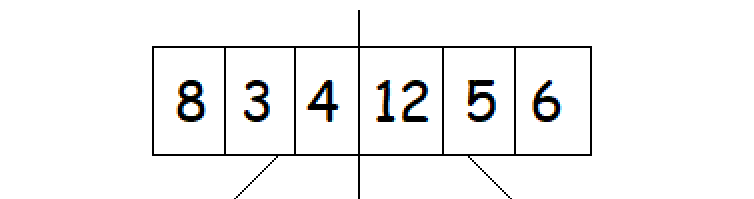2. Again we will find middle index of each part and then divide each part into subsequent sub-arrays.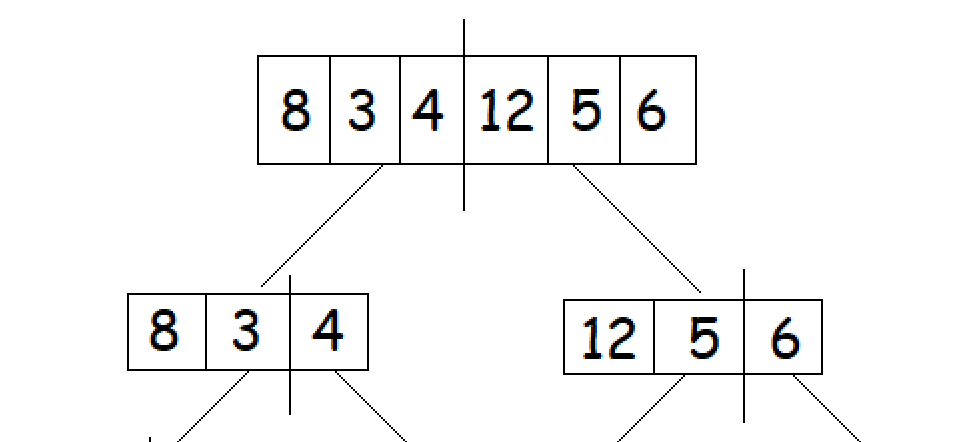3. We will continue splitting the array into halves by finding the middle index of each sub-array unless we reach the atomic value i.e, only single element is left in that particular sub-array.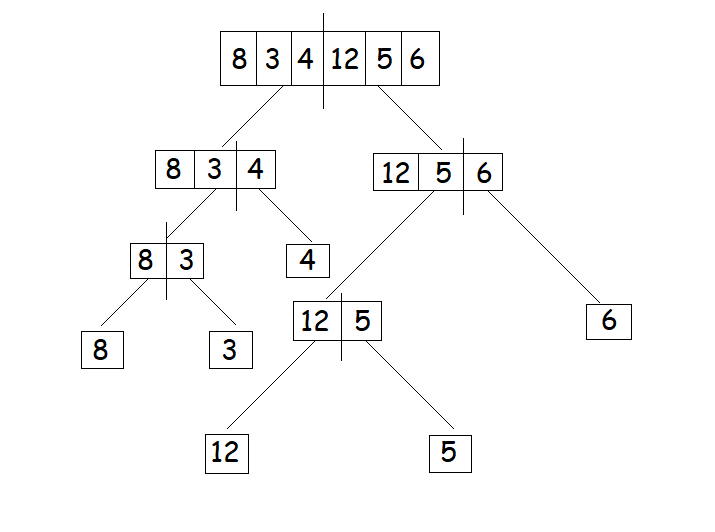4. After that we will start merging the smallest sub-arrays in the sorted manner or based on comparison of size of elements.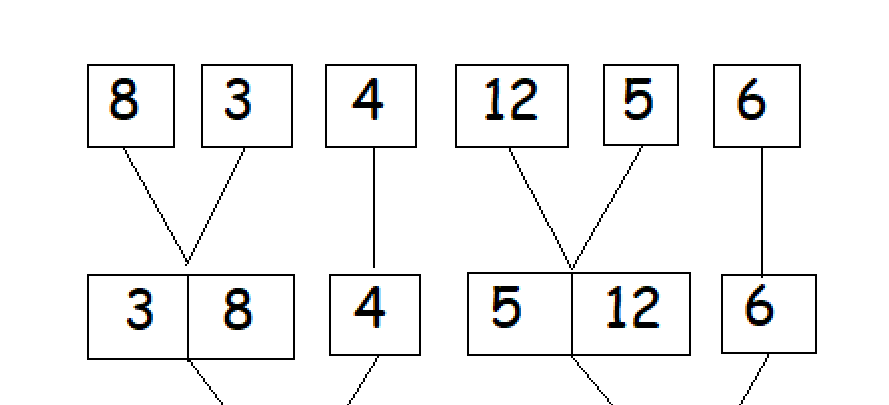5. Finally, after merging each sub-array aur final result will look like this: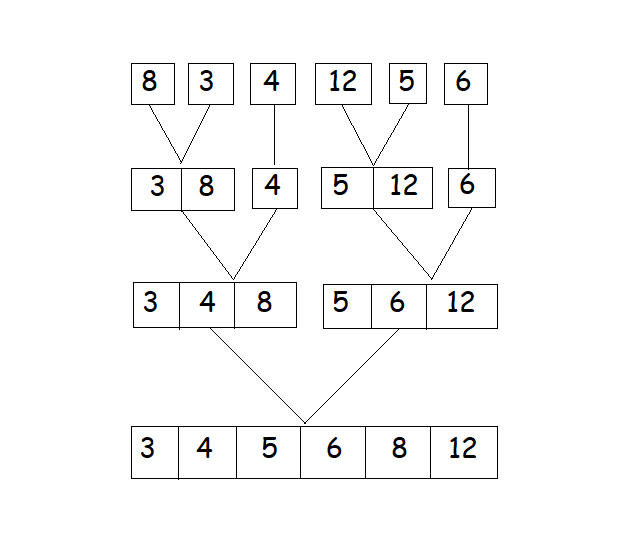Algorithm of Merge Sort:
mergeSort(arr, start, end)

if size > 1

• Find the middle index of input array so that we can divide the array into two-part
• mid = arr.length / 2;
• Call the mergesort for the leftSubArray
• mergeSort(left, 0, mid);
• Call the mergesort for the rightSubArray
• mergeSort(right, mid, arr.length);
• Call the merge function to merge these two halves
• mergeTwoSortedArray(left, right)

## When does worst case occur?

Time complexity of merge sort will depend on the number of comparisons made while merging the sub-arrays in sorted manner. Therefore, we can only improve time complexity if the number of comparisions can be reduced. However there is one case in which we cannot optimise the time complexity and that particular case will come when both left and right sub-arrays will contain alternate numbers.

Let's take an example to make it more clear:

consider, left sub-array as {1,3,5,7} and right sub-array as {2,4,6,8}

In this particular example every element for both arrays needs to be compared at least once to get merged in sorted manner.so, there will be n (total number of elements in input array) comparisions made in merging the sub-arrays. This will be the worst case and will create worst case time complexity of merge sort.

Now, consider a sorted set of numbers [0, 1, 2, 3, 4, 5, 6, 7]:

``````         [4, 0, 6, 2, 5, 1, 7, 3]
/  \
/    \
[4, 0, 6, 2]    [5, 1, 7, 3]
/ \           / \
/   \         /   \
[4, 0] [6, 2]   [5, 1] [7, 3]
|     |        |     |
|     |        |     |
[0, 4] [2, 6]  [1, 5] [3, 7]
\     /        \     /
\   /          \   /
[0, 2, 4, 6]    [1, 3, 5, 7]
\             /
\           /
[0, 1, 2, 3, 4, 5, 6, 7]
``````

Note: at each step, the left and right sub-arrays contain alternate numbers.

So, if the sorted list is [0, 1, 2, 3, 4, 5, 6, 7], then the worst case input for Merge Sort is [4, 0, 6, 2, 5, 1, 7, 3].

`````` [0, 2, 1, 3]
/     \
/       \
[0, 2]  [1, 3]
\       /
\     /
[0, 1, 2, 3]
``````

So, for a sorted list of [0, 1, 2, 3], the worst case input for Merge Sort will be [0, 2, 1, 3].

## Worst Case Time complexity Analysis of Merge Sort

We can divide Merge Sort into 2 steps:

1. Dividing the input array into two equal halves using recursion which takes logarithmic time complexity ie. log(n), where n is number of elements in the input array.
Let's take T1(n) = Time complexity of dividing the array
T1(n) = T1(n/2) + T1(n/2)
T1(n) = 2*T1(n/2)

2. Merging back the divided array into single array in sorted manner by comparing each element of sub-arrays. so, n-1 (number of elements in input array) number of comparsions will took place in merging the sub-arrays in the worst case as discussed above.

Let's take T2(n) = Time complexity in merging the sub-arrays
T2(n) = n-1

Therefore, Total time complexity of merge sort = T1(n) +T2(n)
Let's take T(n) = Total time complexity of merge sort
T(n) = 2*T(n/2) + n-1

Using Akra Bazzi formula to solve above recurrance relation: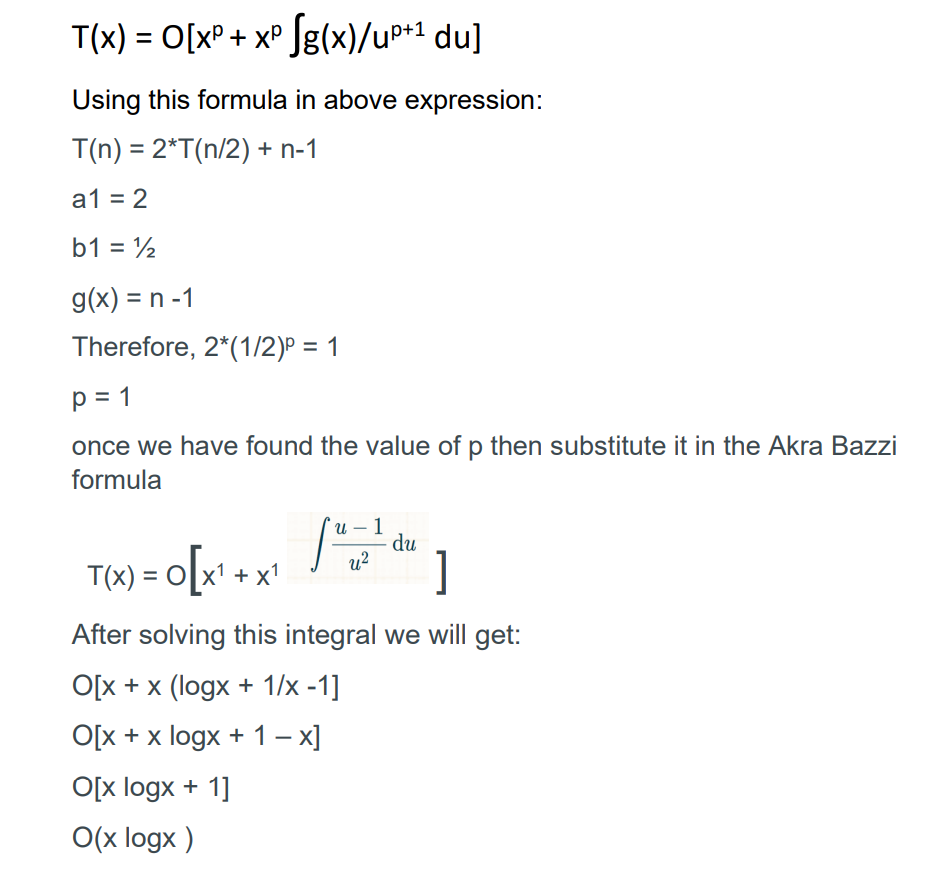So, for given array of size n time complexity of merge sort will be O(nlogn).

## Implementation of Merge Sort

As we already know Merge Sort is based on divide and conquer sorting algorithm.
So, given below is the implementation of Merge sort in java programming language:

Pseudo Code of Merge Sort:

``````mergeSort(int[] arr)

1. Find the middle point to divide the input into two halves:
middle = arr.length/2
2. Call MergeSort for first half:
int[] left = mergeSort(Arrays.copyOfRange(arr, 0, mid));
3. Call MergeSort for the other half:
int[] rigth = mergeSort(Arrays.copyOfRange(arr, mid, arr.length));
4. Merge the two halves sorted in step 2 and 3:
Call Merge(left, right)

``````

Code:

``````import java.util.Arrays;

public class Merge_Sort {
public static void main(String[] args) {
int[] arr = {5, 4, 3, 2, 1};
arr = mergeSort(arr);
System.out.println(Arrays.toString(arr));
}
static int[] mergeSort(int[] arr) {
if (arr.length == 1) {
return arr;
}

int mid = arr.length / 2;

int[] left = mergeSort(Arrays.copyOfRange(arr, 0, mid));
int[] right = mergeSort(Arrays.copyOfRange(arr, mid, arr.length));

return merge(left, right);
}

private static int[] merge(int[] first, int[] second) {
int[] mix = new int[first.length + second.length];

int i = 0;
int j = 0;
int k = 0;

while (i < first.length && j < second.length) {
if (first[i] < second[j]) {
mix[k] = first[i];
i++;
} else {
mix[k] = second[j];
j++;
}
k++;
}

// it may be possible that one of the arrays is not complete
// copy the remaining elements
while (i < first.length) {
mix[k] = first[i];
i++;
k++;
}

while (j < second.length)
{
mix[k] = second[j];
j++;
k++;
}

return mix;
}

}
``````

Output: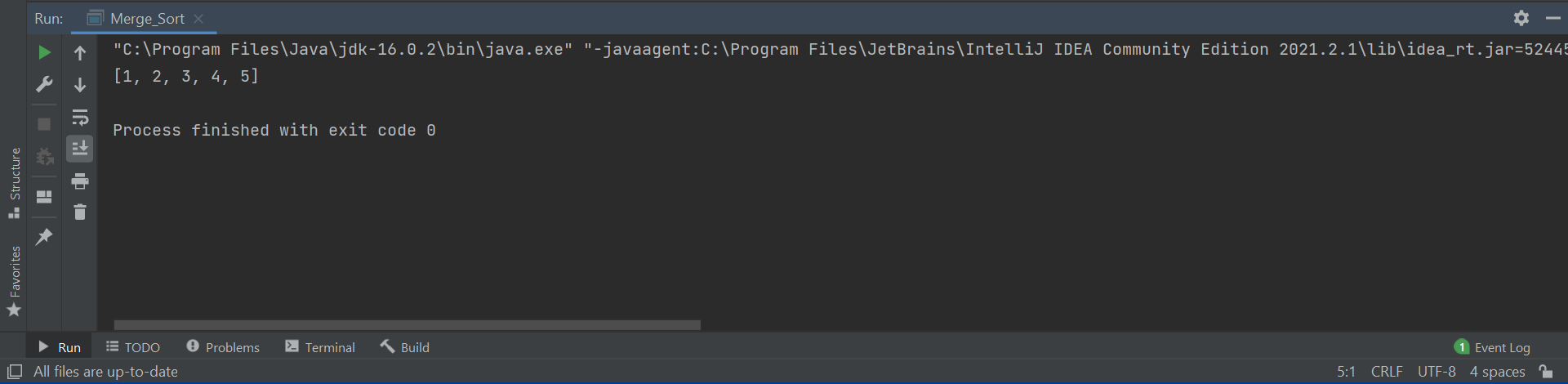Here, we can see that an sorted array is being displayed in output window.

## Conclusion:

In this article, I have discussed about Merge sort, its worst case time complexity which is O(nlogn) where n is input size of array. I have also discussed when will worst case occur i,e: when both left and right sub-arrays will contain alternate numbers.

#### Uddeshya Raj

Uddeshya Raj is pursuing B. Tech in Computer Science from Chandigarh University. He is an Algorithm and Data Structure Developer, Intern at OpenGenus.